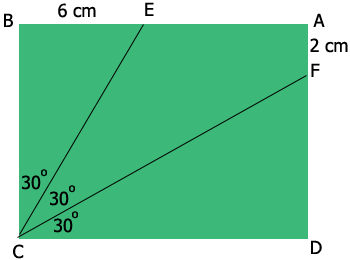SEARCH HOMEMath Central Quandaries & QueriesQuestion from Ahamed: In rectangle ABCD,angle BCD is trisected. CE,CF meet the sides AB,AD at E,F. BE=6cm,AF=2cm so, find the area of the rectangle ABCD?Hi,

Here is my diagram,First consider triangle $EBC.$ Angle $EBC$ is a right angle and angle $BCE$ is one third of angle $BCD$ so the measure of angle $BCE$ is $30^{o}.$ What is the length of $BC?$

Now consider triangle $FCD.$

PennyMath Central is supported by the University of Regina and The Pacific Institute for the Mathematical Sciences.